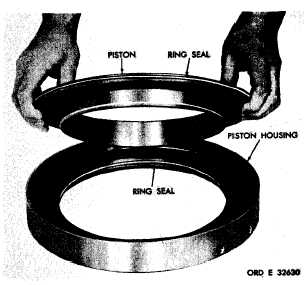Custom SearchC H A P    5,   SEC   X X II C L U T C H    P I S T O N S    A N D    H O U S I N G S    R E B U I LD PAR  3 - 1 7 6 ( 2 )    C h e c k    t h e    s m a l l    h o l e    t h r o u g h    t he p i s t o n. It   must   be   clean. .   I n t e r m e d i a t e - r a n g e    P i s t o n    a nd Housing   (fig.   211) ( 1 )     R e m o v e     t h e     i n t e r m e d i a t e - r a n g e piston   from   the   piston   housing. (2)   Remove   ring   seal   and   expander   from the   piston. (3)   Remove   ring   seal   and   expander   from the   piston   housing. 1 7 4 .     C L E A N I NG Refer   to   par.   71   for   cleaning   recommen- d a t i o n s. Figure   211.   Removing   (or   installing)   intermediate- 1 7 5 .    I N S P E C T I O N    A N D    R E P A IR range piston Refer   to   par.   72   for   general   information and    repair    recommendations.    Repair    and    re- (1)   Install   expander   60   (fig.   377,   fold- b u i l d    p o i n t s    o f    m e a s u r e m e n t    f o r    f i t s ,    c l e a r - out   6)   and   ring   seal   59   onto   the   steer   clutch antes   and   wear   limits   are   indicated   by   small, piston   61. lower   case   letters   in   figs.   376,   377   and   381, f o l d - o u t s    5 ,    6    a n d    1 0 .    R e f e r    t o    p a r s .    2 3 9, (2)   Install   expander   58   and   ring   seal   57 240   and   244   for   wear   limits   information. onto   piston   61. 176.   ASSEMBLY   (figs.   376,   377   and   381, fold-outs   5,   6   and   10) .   I n t e r m e d i a t e - r a n g e    P i s t o n    a n d Housing   (fig.   376,   fold-out   5) (1)   Install   ring   seal   12   and   expander   13 .   R i g h t -    o r    L e f t - s t e e r    C l u t ch in   the   intermediate-range   piston   housing   14. P i s t o n     A s s e m b ly (2)   Install   expander   10   and   ring   seal   11 .   T h e    r i g h t -    a n d    l e f t - s t e e r    c l u t c h onto   the   piston. p i s t o n    a s s e m b l i e s    a r e    i d e n t i c a l .    T h e following   describes   the   assembly   of   the (3)    Install    the    intermediate-range    piston l e f t    a s s e m b l y. into   the   piston   housing   14. 1 4 6